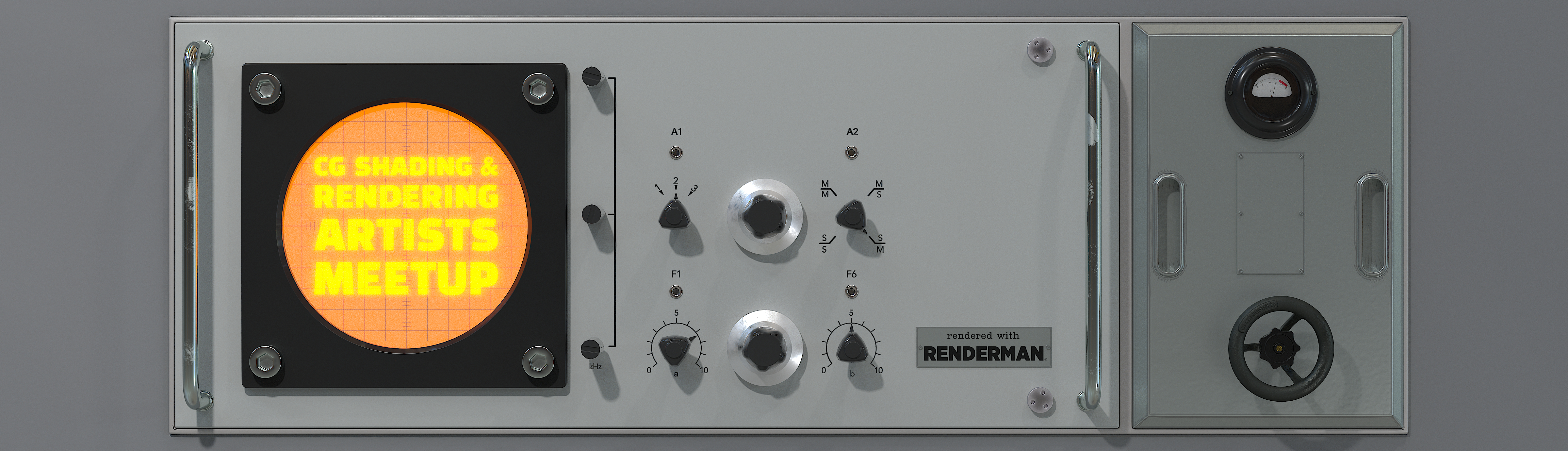# haki

Members

8

## Community Reputation

5 Neutral

• Rank
Peon
• Birthday 06/25/1988

## Contact Methods

• Website URL
https://hristo.one

## Personal Information

• Name
• Location
The Netherlands
1. Hey folks, back with an update here, I took care of the curve framing: Thanks to Entagma's Parallel Transport tutorial I understood the core concept here. I adapted it to my curves generator. I tried 2-3 alternatives, including the slideframe() function and the dihedral()*v0. Seems I got the best result by crossing vectors only. The slideframe and the dihedral seemed somewhat off, probably because I didn't do something right. Here's the file if you wanna give it try: rand_dir_propagating_points_3.hiplc
2. Eureka! (No need for point arrays after all...) So, from what I originally intended to be a grass generator... it will be a multi-purpose generator (including the effect on hair that electrocution has according to popular cartoon depictions) Still to figure out a way to control the up angle at every sample... and to orient the points correctly based on the starting points (polyframe the prims I guess...)  - With some finetuning below: rand_dir_propagating_points_2.hiplc
3. Ta-da! I got the point arrays exactly in the correct order for the point number pattern I was getting. Alright, it's going to be smooth sailing from here on… (doing a little dance) Wait, what!? Addprim didn't do what you imagined? Documentation, here I come.
4. Cheers, @Librarian I hope you can find some use for it. I attached the current WIP file there. I can't wrap my head around how to gain manual control over the rand vector for each sample yet.
5. A quick update: rand_dir_propagating_points.hiplc
6. Thanks, Thomas! Ok. It seems I'm missing something here to understand why it always gets (0,0,0). On sample 1 it doesn't seem to get (0,0,0). Let me see. Maybe my comment's complete nonsense, so back to the drawing board: It seems like this does what I've sketched above: Now onto figuring out how to gain some degree of manual control over the random angle for each sample... Cheers!
7. Cheers, Thomas! That explains some of the confusion I had. And, you're right I was probably overthinking it. Thanks for the base script! I didn't write that in the post above, but I intended to later randomize the up vector direction for every new point and thus get a non-linear propagating pattern. I want to achieve a sort of (and I suspect that's not the right term here) parent-child behavior between every point and the next in line (something like tentacles constructed by points propagating in random directions). That's the reason why (in my head at least), calculating a new up by subtracting current_pos from new_pos and setting that at every sample is important. Except, and this is probably going to seem totally dumb... at sample 2 it falls apart. Up is no longer pointing where I'd expect it to. I bet this has something to do with the last line current_pos = point(0, "P", new_point); rand_dir_propagating_points.hiplc vector up = @up; vector current_pos = @P; vector new_pos = 0; int samples = chi("samples"); float offset = chf("offset"); for(int i = 0; i < samples; i++){ new_pos += current_pos + up * offset; int new_point = addpoint(0, new_pos); up = normalize(new_pos-current_pos); setpointattrib(0, "up", new_point, up, "set"); current_pos = point(0, "P", new_point); } It appears at sample 2, the up direction is pointing away from the world origin, and not away from the points at sample 1. I feel like I'm missing something so basic...
8. LATEST WIP UPDATE: ---------------------------------------------------------------------------------------------------------------------------------------------------- Hi folks, How would you go about adding points in a propagating manner in VEX. Here's what I mean: - Start with several incoming base points, each with an up vector. - For each one, add a new point somewhere along its up vector. - Calculate a new up vector by subtracting base positions from new point positions and setting the new point up vector to the result - Add the next new point somewhere along the previous point's up vector So, I called it propagating, because every next point in part defines the following. I think I'm on the right track here, but my gut's telling me I'm missing something. I'm stuck. Here's what I got: vector up = point(0, "up", @ptnum); vector currentPos = point(0, "P", @ptnum); vector newPos = {0,0,0}; int pts[]; for(int i=0; i<@numelem; i++) { append(pts,i); } int iteration = chi("iteration"); for(int j=0; j<=iteration-1; j++){ newPos = currentPos+up; int newPoint = addpoint(0, newPos); setpointattrib(0, "up", len(pts)+j, normalize(newPos), "set"); currentPos = point(0, "P", len(pts)+j); up = normalize(newPos-currentPos); currentPos = newPos; } Where do you think I'm wrong here. What would you do? Cheers!
×
• Donations